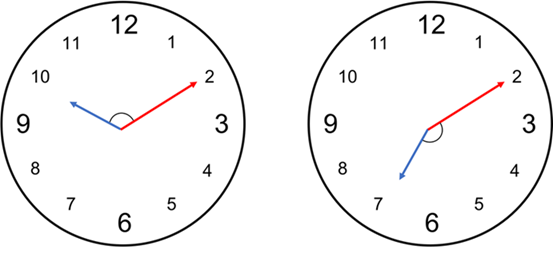# Problem 56288. Angle Between Analog Clock Hands

Given a datetime variable t, return the angle (in degrees) between the hour and minute hands of an analog clock at the time represented by t.• The angle returned should be the smaller of the two angles between the hands (ie it should always be in the range [0,180]).
• Assume the hands are moving continuously (so at 03:30:00, the hour hand will be halfway between 3 and 4; at 03:26:30, the minute hand will be halfway between 26 and 27, etc).
• The time may be am or pm; the angle returned should be assuming a standard 12-hour clock (so 03:26:30 and 15:26:30 will return the same angle).

### Solution Stats

29.18% Correct | 70.82% Incorrect
Last Solution submitted on Dec 03, 2023

### Community Treasure Hunt

Find the treasures in MATLAB Central and discover how the community can help you!

Start Hunting!